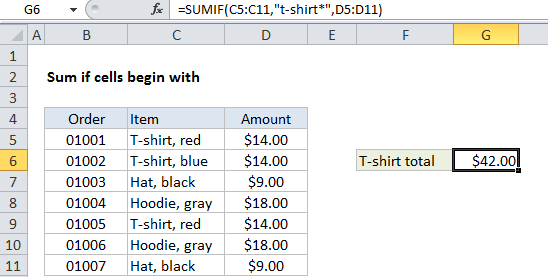## Excel Office

Excel How Tos, Tutorials, Tips & Tricks, Shortcuts

# Sum if begins with in Excel

This tutorial shows how to Sum if begins with in Excel using the example below;

## Formula

`=SUMIF(range,"text*",sum_range)`## Explanation

To sum cells if other cells begin with a specific value, you can use the SUMIF function.

In the example shown, cell G6 contains this formula:

`=SUMIF(C5:C11,"t-shirt*",D5:D11)`

This formula sums the amounts in column D when a value in column C begins with “t-shirt”. Note that SUMIF is not case-sensitive.

Also See:   Sum if equal to either x or y in Excel

### How the formula works

The SUMIF function supports wildcards. An asterisk (*) means “one or more characters”, while a question mark (?) means “any one character”.

These wildcards allow you to create criteria such as “begins with”, “ends with”, “contains 3 characters” and so on.

To match all items that begin with “T-shirt”, the criteria is “t-shirt*”. Note that you must enclose literal text and the wildcard in double quotes (“”).

Also See:   SUMIFS function: Description, Usage, Syntax, Examples and Explanation

### Alternative with SUMIFS

You can also use the SUMIFS function to sum if cells begin with. SUMIFS can handle multiple criteria, and the order of the arguments is different from SUMIF. The equivalent SUMIFS formula is:

`=SUMIFS(D5:D11,C5:C11,"t-shirt*")`

Notice that the sum range always comes first in the SUMIFS function.